# Temperature Coefficient Of Resistance

Usually, the temperature affects the resistance and electrical resistivity of all materials. Further, when there is a change in electrical resistance it has a great bearing on different electrical and electronic circuits. There could also be instances where we will witness significant changes. Due to this factor, the temperature coefficient of resistance is an important topic that we should understand in many electrical applications.

## What is Temperature Coefficient of Resistance?

The temperature coefficient of resistance is generally defined as the change in electrical resistance of a substance with respect to per degree change in temperature.

So if we look at the electrical resistance of conductors such as gold, aluminium, silver, copper,  it all depends upon the process of collision between the electrons within the material. When the temperature increases, the process of electron collision becomes rapid and faster. As a result, the resistance will increase with the rise in temperature of the conductor.

## Relation between Temperature and Resistances

Let us consider a conductor whose resistance at 0°C is R0 and the resistance at a temperature T°C is RT. The relation between temperature and resistances R0 and RT is approximately given as

RT = R0 [1+ α (T-T0)];

RT = R0 [1+ α (∆T)]

Hence it is clear from the above equation that the change in electrical resistance of any substance due to temperature depends mainly on three factors –

1. The value of resistance at an initial temperature.
2. The rise in temperature.
3. The temperature coefficient of resistance α.

The value of α can vary depending on the type of material. In metals, as the temperature increases the electrons attain more kinetic energy, thus more speed to undergo frequent collisions. We know that the resistivity of any substance is given by

ρ = m/nq2 τ

So, the resistivity depends on the number of charge carriers per unit volume n and the relaxation time τ between collisions. When the temperature of the metal is increased, the average velocity of the current carriers i.e the electrons increases and result in more collisions.

This means that the average time between successive collisions τ decreases. But the change in the value of n due to the increase in temperature is negligible which further means that the value of resistivity now is dependent only on the change in τ.

## Types of Temperature Coefficient Of Resistance

There are two main types of temperature coefficient of resistance.

### Positive Temperature Coefficient Of Resistance

The resistivity and the resistance of the material increases due to decrease in τ. Hence the value of the temperature coefficient of metal is positive.

### Negative Temperature Coefficient Of Resistance

In the case of semiconductors and insulators, the number of charge carriers per unit volume n increases with an increase in temperature. The decrease in τ is compensated well by the increase in n such that the value of resistivity and resistance decreases with an increase in temperature.

Hence, the value of the temperature coefficient of resistivity in semiconductors and insulators is negative. The temperature dependence of resistance in conductors, semiconductors and insulators are represented in figure 1 and figure 2.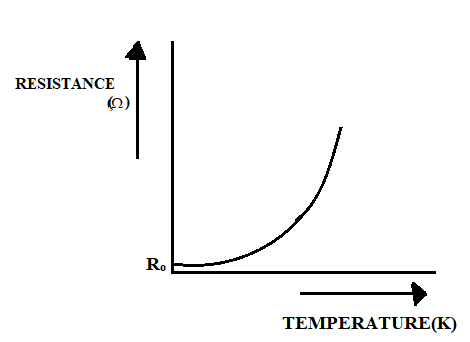The curve in figure 1 shows that the value of resistance increases with an increase in temperature. The value of resistance at zero units of temperature is represented as R0.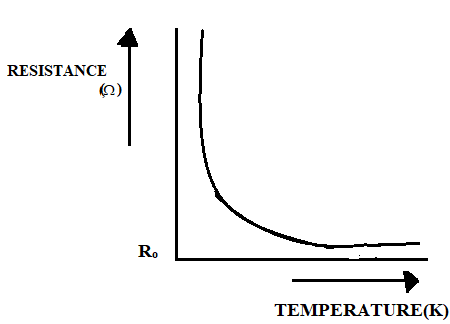The curve in figure 2 represents the typical nature of the resistance of a semiconductor as a function of temperature.

 Material Temperature coefficient of resistance / 0C (at 200 C) Iron (Fe) 0.00651 Aluminium (Al) 0.00429 Gold (Au) 0.0034 Silver (Ag) 0.0038 Platinum (Pt) 0.003927 Copper (Cu) 0.00386 Tin (Sn) 0.0042 Tungsten (W) 0.0045 Silicon (Si) – 0.07 Brass 0.0015 Nickel (Ni) 0.00641 Mercury (Hg) 0.0009

### Solved Questions

Question 1: The resistance of a wire is 5Ω at 50°C and 6Ω at 100°C. The resistance of the wire at 0°C will be? [AIEEE 2007]

Solution:

We know that RT = R0 [1+ α (T-T0)]

5= R0 [1+50α]………(1)

6 = R0 [1+100α]……..(2)

Dividing equation (1) and (2)

5/6 = 1+50α/1+100α

α = 1/200

from (1)

5 = R0 [1+50(1/200)]

R0 = 4Ω

Question 2: The resistance of a bulb filament is 100Ω at a temperature of 100°C. If its temperature coefficient of resistance be 0.005 per °C, its resistance will become 200Ω at a temperature of? [AIEEE 2006]

Solution:

As we know that RT = R0 [1+ α (T-T0)]

100 = R0 [1+ 0.005×100]

And 200 = R0 [1+ 0.005x T]

Here T is the temperature in °C at which the resistance becomes 200Ω.

200/100 = (1+ 0.005x T)/(1+ 0.005×100)

T= 400°C

Question 3: A platinum resistance thermometer has a resistance R0 = 40.0 Ω at T0=30 ºC. α for Pt is 3.92×10-3(ºC)-1. The thermometer is immersed in a vessel containing melting tin, at which point R increases to 94.6Ω. What is the melting point of tin?

Given:

R0 = 40.0 Ω, RT = 94.6Ω

T0=30 ºC, T = ?

Solution:

RT = R0 [1+ α (T-T0)]

94.6Ω = 40Ω [1 + 3.92 ×10-3 (ºC)-1 (T–30ºC)]

2.365 = [1 + 3.92 ×10-3 (ºC)-1 (T–30ºC)]

1.365 = 3.92 ×10-3 (ºC)-1 (T–30ºC)

211ºC = T–30ºC

T = 241 ºC

The melting point of tin is 2410C.

## Kirchoff’s Rules

Kirchoff’s current rule also called Junction rule states that “at any junction, the sum of the currents entering the junction is equal to the sum of the currents leaving the junction.”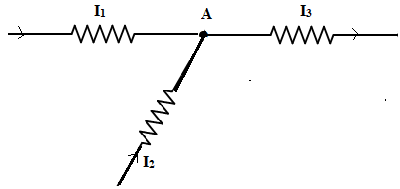At junction A in figure 3, the current entering = I1 + I2

The current leaving = I3

By applying Kirchoff’s junction rule, we can say that I1 + I2 = I3

Also Read: Ohm’s Law and Resistance

This rule can be applied to a junction as well as to a point on a line. This rule is inferred from the assumption that there is no accumulation of charges at any junction or point on the current-carrying wire, therefore the total current that flows in should be equal to the total current that flows out.

### Kirchoff’s Voltage Rule or Loop Rule

The rule states that “the algebraic sum of changes in potential around any closed loop involving resistors and cells in the loop is zero.” An electrical potential is a quantity dependant on the location of the point. So in a closed-loop, if we start from a point and come back to the same point, the total change in potential should be zero. This rule is based on the law of conservation of energy.

For a closed-loop  Ʃ∆V = 0

Ʃ∆E + RI = 0

In other words, the sum of the all EMFs and product of the currents and resistances in a closed loop is zero.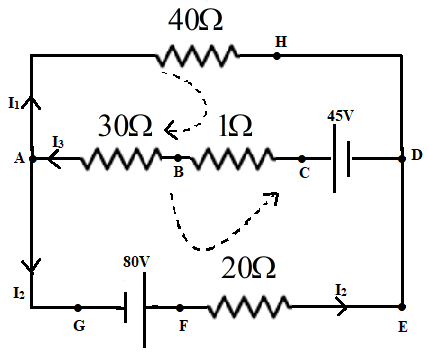Let us consider a circuit as represented in the figure given above to explain how the loop rule is applied. At junction A the currents leaving are I1 + I2 while is I3 is entering. At the junction, current entering is I1. Hence by applying junction rule the current leaving should also be equal to I1.

Let us consider the loop ‘AHDCBA’. Applying the loop rule we get,

-40 I1 – 31 I3 + 45= 0.

Similarly, if we applying loop rule to the loop ‘AHDEFGA’ gives us

-40 I1 + 21 I2 + 80 = 0

Kirchoff’s rules can be used to simplify complicated networks involving many numbers of electrical components and multiple junctions where calculations using simple ideas of series and parallel connections fail to provide desired results.

### Solved Questions

Question 1: Find the value of current I in the circuit given below.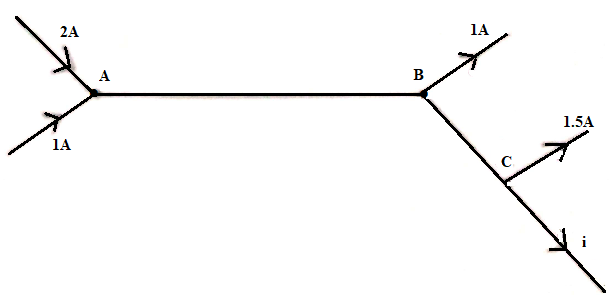Solution: Apply Kirchoff’s junction rule at junction A, B and C. As per junction rule the sum of the currents entering is equal to the currents leaving.

At junction A

2A + 1A = 3A

A current of 3A flows through the wire of length AB

At junction B, the current leaving through BC can be found out as = 3A -1A

= 2A

At junction C, the current i is calculated as

2A = 1.5A+ i

i = 0.5A

So the unknown current i = 0.5A

Question 2: Determine the current through the given electrical circuit if R1 = 4Ω, R2 = 8Ω and R3 = 12Ω.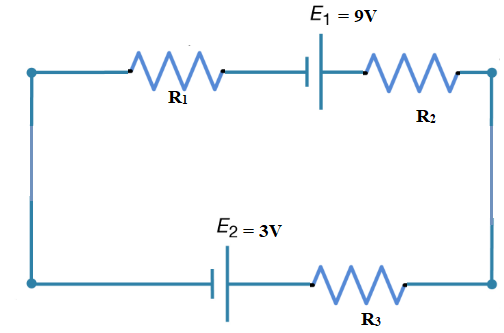Solution: Firstly, let us choose the direction of the current to be in a clockwise direction. Whenever a current flows through a resistor, there is a potential drop, hence it is taken with a negative sign.

If the current flows from low to high voltage( – to +) then the source of emf E is taken positively as this is considered as the charging of energy at the emf source. If the current flows from high to low voltage (+ to -) then the source of emf E is taken negatively as this is considered as the discharging/emptying of energy at the emf source.

Now applying Kirchoff’s loop rule,

-I R1 + E1 -I R2 – I R3 – E2 = 0

-4I + 9 – 8I – 12I – 3 = 0

24I = 6

I = ¼ = 0.25A

Hence, the electric current flowing in this circuit is 0.25 A. Since our calculated answer for current is positive, this implies that our initial assumption of the direction of current is correct. If the electric current is calculated to be negative, this implies that the initial assumption for the direction of the current should be anti-clockwise.

3. Calculate the terminal voltage of the battery in the circuit below?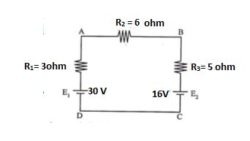Given:

R1 = 3 Ω

R2 = 6 Ω

R3 = 5 Ω

emf 1 (E1) = 30 V

emf 2 (E2) = 16 V

Terminal Voltage (V) is the potential difference between the terminals when battery current flows out.
If the battery does not draw any current, the terminal voltage equals the emf.

Terminal Voltage (V) = E– E2

V = 30 V – 16 V

V = 14 V